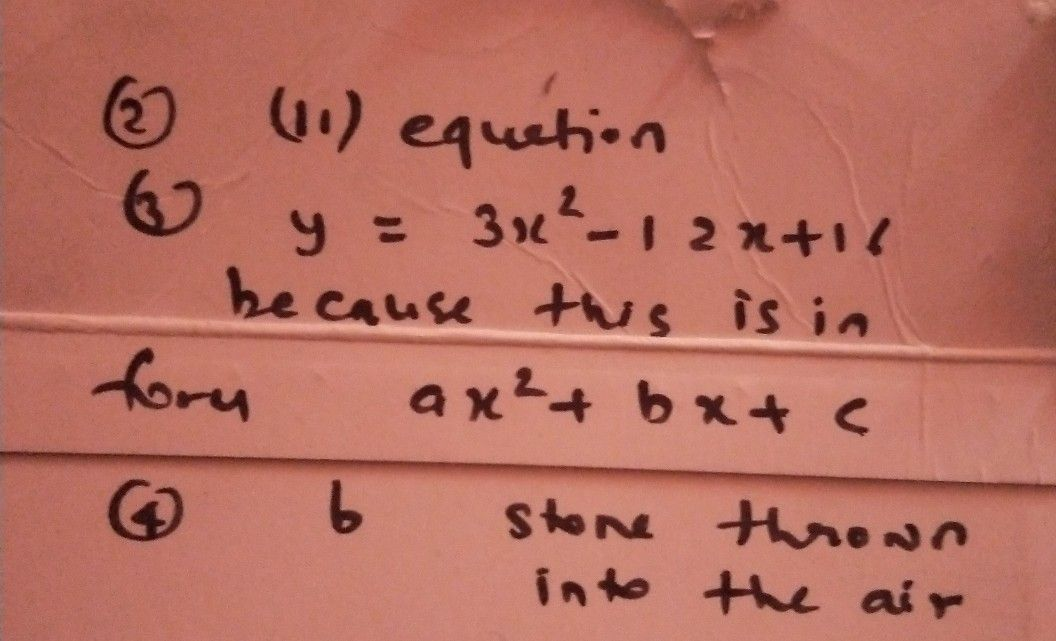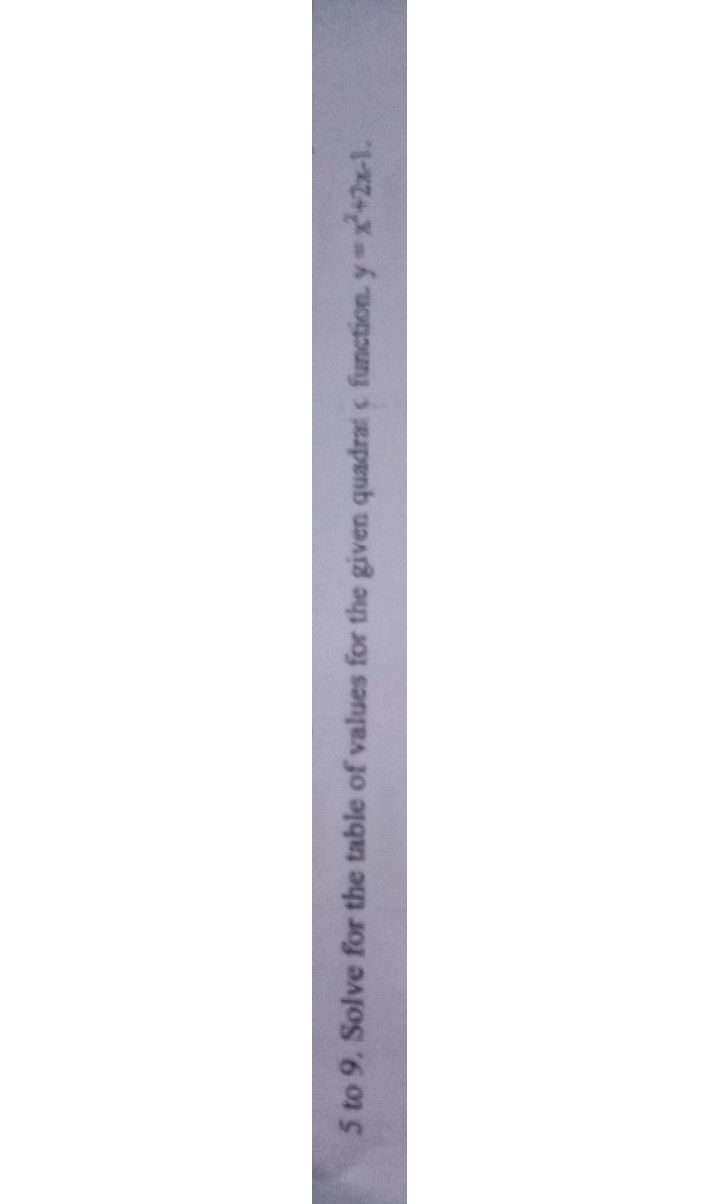Symbol
Problem2. Quadratic function can be represented $11sing$ $i.$ graph ii. equation iii. $1\pi csian$ $1V$ table of values $a1ucs$ a. i only b. i and ii $C.$ i, ii, $an0$ iii d. i, ii, $an0$ iv $3.$ Which of the following is an example of quadratic $\bar{5} 1n$ ion? $n7$ $a.y=x^{3}+7x$ $b.y=3^{x}-4x+2$ $c.y=3x^{2}-12x+16$ d. $x+7$ 4. The following models the quadratic function in real li e $e\times cept$ a. high energy $eVe$ b. stone thrown into the ai c. $ba1|$ launched upward d. fired vertically upward
7th-9th grade
Other
SolutionQanda teacher - nitinpearlStudent
Thank you very much Mr. Nitinpearl